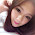## Wednesday, July 29, 2009

### Wilcoxon signed rank test

Non-parametric statistical hypothesis test, for the comparison of the means between 2 paired samples

The mayor of a city wants to see if pollution levels are reduced by closing the streets to the car traffic. This is measured by the rate of pollution every 60 minutes (8am 22pm: total of 15 measurements) in a day when traffic is open, and in a day of closure to traffic. Here the values of air pollution:

With traffic: 214, 159, 169, 202, 103, 119, 200, 109, 132, 142, 194, 104, 219, 119, 234
Without traffic: 159, 135, 141, 101, 102, 168, 62, 167, 174, 159, 66, 118, 181, 171, 112

It is clear that the two groups are paired, because there is a bond between the readings, consisting in the fact that we are considering the same city (with its peculiarities weather, ventilation, etc.) albeit in two different days. Not being able to assume a Gaussian distribution for the values recorded, we must proceed with a non-parametric test, the Wilcoxon signed rank test.

`a <- c(214, 159, 169, 202, 103, 119, 200, 109, 132, 142, 194, 104, 219, 119, 234)b <- c(159, 135, 141, 101, 102, 168, 62, 167, 174, 159, 66, 118, 181, 171, 112)wilcox.test(a,b, paired=TRUE)Wilcoxon signed rank testdata: a and bV = 80, p-value = 0.2769alternative hypothesis: true location shift is not equal to 0`

Since the p-value is greater than 0.05, we conclude that the means have remained essentially unchanged (we accept the null hypothesis H0), then blocking traffic for a single day did not lead to any improvement in terms of pollution of the city.
The value `V = 80` corresponds to the sum of ranks assigned to the differences with positive sign. We can manually calculate the sum of ranks assigned to the differences with positive sign, and the sum of ranks assigned to the differences with negative sign, to compare this interval with the interval tabulated on the tables of Wilcoxon for paired samples, and confirm our statistic decision. Here's how to calculate the two sums.

`a <- c(214, 159, 169, 202, 103, 119, 200, 109, 132, 142, 194, 104, 219, 119, 234)b <- c(159, 135, 141, 101, 102, 168, 62, 167, 174, 159, 66, 118, 181, 171, 112) diff <- c(a - b) #calculating the vector containing the differences diff <- diff[ diff!=0 ] #delete all differences equal to zero diff.rank <- rank(abs(diff)) #check the ranks of the differences, taken in absolute diff.rank.sign <- diff.rank * sign(diff) #check the sign to the ranks, recalling the signs of the values of the differences ranks.pos <- sum(diff.rank.sign[diff.rank.sign > 0]) #calculating the sum of ranks assigned to the differences as a positive, ie greater than zero ranks.neg <- -sum(diff.rank.sign[diff.rank.sign < 0]) #calculating the sum of ranks assigned to the differences as a negative, ie less than zero ranks.pos #it is the value V of the wilcoxon signed rank test 80 ranks.neg 40`

The computed interval is (40, 80). The tabulated interval on Wilcoxon paired samples tables, with 15 differences, is (25, 95). Since the calculated interval is contained in the tabulated, we accept the null hypothesis H0 of equality of the means. As predicted by the p-value, closing roads to traffic did not bring any improvement in terms of rate of pollution.

1.2.